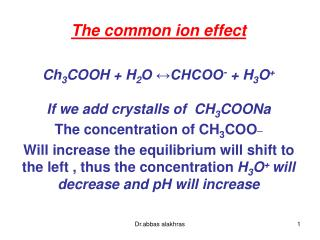Download PresentationThe common ion effect Ch 3 COOH + H 2 O ↔CHCOO - + H 3 O + If we add crystalls of CH 3 COONa

Loading in 2 Seconds...

# The common ion effect Ch 3 COOH + H 2 O ↔CHCOO - + H 3 O + If we add crystalls of CH 3 COONa - PowerPoint PPT Presentation

The common ion effect Ch 3 COOH + H 2 O ↔CHCOO - + H 3 O + If we add crystalls of CH 3 COONa The concentration of CH 3 COO _ Will increase the equilibrium will shift to the left , thus the concentration H 3 O + will decrease and pH will increase. Example:I am the owner, or an agent authorized to act on behalf of the owner, of the copyrighted work described.
Download Presentation## The common ion effect Ch 3 COOH + H 2 O ↔CHCOO - + H 3 O + If we add crystalls of CH 3 COONa

An Image/Link below is provided (as is) to download presentation

Download Policy: Content on the Website is provided to you AS IS for your information and personal use and may not be sold / licensed / shared on other websites without getting consent from its author.While downloading, if for some reason you are not able to download a presentation, the publisher may have deleted the file from their server.

- - - - - - - - - - - - - - - - - - - - - - - - - - E N D - - - - - - - - - - - - - - - - - - - - - - - - - -
Presentation Transcript
1. The common ion effect Ch3COOH + H2O ↔CHCOO- + H3O+ If we add crystalls of CH3COONa The concentration of CH3COO_ Will increase the equilibrium will shift to the left , thus the concentration H3O+ will decrease and pH will increase Dr.abbas alakhras

2. Example: a) what is the pH of 0.03 Ch3COOH Ka Ch3COOH = 1.8x10-5 b) What will be the pH if 0.03 M CH3COOH is added . To the above Solution : Ch3COOH + H2O ↔CHCOO- + H3O+ 0.3-x مهمله)) x x Ka = x2/ 0.3 = 1.8x10-5 X= 2.3x 10-3 M = [H+] then then PH=2.63 Dr.abbas alakhras

3. b)Ch3COOH + H2O ↔CHCOO- + H3O+ 0.3 البدايه) (0.3 0.3-x مهمله)) 0.3+ x x مهمله Ka = 0.3 (x) / 0.3 = 1.8x10-5= (H+) pH= 4.74 Dr.abbas alakhras

4. Example :(important ) page 663 17-2 Calculate the florid ion concentration and PH of a solution that is 0.2M in HF and 0.1 M in HCl Dr.abbas alakhras

5. Solution [F-] === ??? pH=== ???? HCl= 0.1 mol HF = 0.2 mole V= 1.0 L Ka HF= 6.8x10-4 [HCl] = 0.1/ 1= 0.1 M [HF] = 0.2/ 1= 0.2 M HF + H2O ↔ H+ + F- 0.2 البدايه)) 0.1 zero HCl مصدرها 0.2 – x 0.1+x x Dr.abbas alakhras

6. مصدرها HCL Ka= (0.1)(x)/ 0.2 = 6.8x10-4 X = [F-] = 1.4x10-3M PH= - log H+ = -log 0.1 = 1 Practice page: 664 Dr.abbas alakhras

7. NH3+ H2O ↔ NH4+ + OH- If we add acrystalls of NH4Cl the equilibrium will shift to left , [OH-] ↓ [H+]↑ PH ↓ Dr.abbas alakhras

8. Buffer solution A buffer consist of weak acid its conjugate base or weak base and its conjugate acid • A buffer resist a small charge in PH when strong acid or base is added to the buffer Dr.abbas alakhras

9. Which of the following pairs is not a buffer: HCN/ NaCN ▓ HCl/ NaCl متفرج ( NO) HF/ NaF ▓ HNO3/NO3- متفرج ( NO) CH3COOH/CH3COONa ▓ NH3/NH4Cl ▓ H2CO3/CO3-2ليست مترافقه H2CO3/HCO3- ▓ Dr.abbas alakhras

10. {CH3COOH 0.3M / 0.15 M NaOH} CH3COOH + NaOH→ CH3COONa+ H2O 0.3 0.15 zero Limiting reactant 0.3 – 0.15 0.15 – 0.150.15 Conjugate pair buffer Dr.abbas alakhras

11. Homework Is the following pairs is a buffer: NH3 0.3 M / 0.3 M HCl What is the PH of the a above solution K b NH3= 1.8x10-5 Dr.abbas alakhras

12. Henderson – Hasse lalch equation PH = PKa + log [A-] / [HA] Weak acid Conjugate base PH = PK b + log [HB+] / [B] Weak base Conjugate acid PK b = - log [Kb] Dr.abbas alakhras

13. Dr.abbas alakhras